# Simple angle meter using ADXL335 accelerometer [Arduino]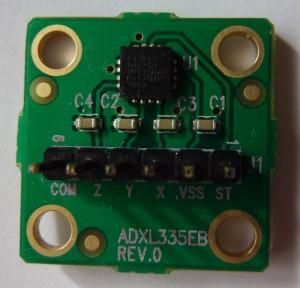ADXL335 is 3 axis accelerometer with analog output from Analog Devices. You can buy it as an evaluation kit  with standard 2,5 mm connector.

ADXL335 acceleration measurement range is +/- 3 g. Supply voltage is 1.8 –  3.6 V, however all specifications at the datasheet is given at 3.0 V. This accelerometer has  3 outputs for X,Y,Z axis which voltage is proportional to acceleration on specific axis.

At midpoint when acceleration is 0 g output is typically 1/2 of supply voltage. If a supply voltage is 3V, then output is 1.5 V. Output sensitivity typically is 300 mV/g.

# Arduino frequency counter/duty cycle meter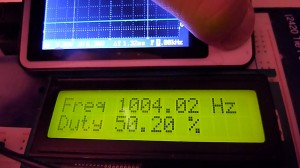This meter gives the best results at 0 – 1000 Hz  range. It works by measuring square wave total and high period duration using 16 bit hardware counter.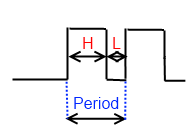As you may know frequency = 1/Period and  Duty Cycle = High period duration/total period duration.

# Arduino GPS clock using NMEA protocol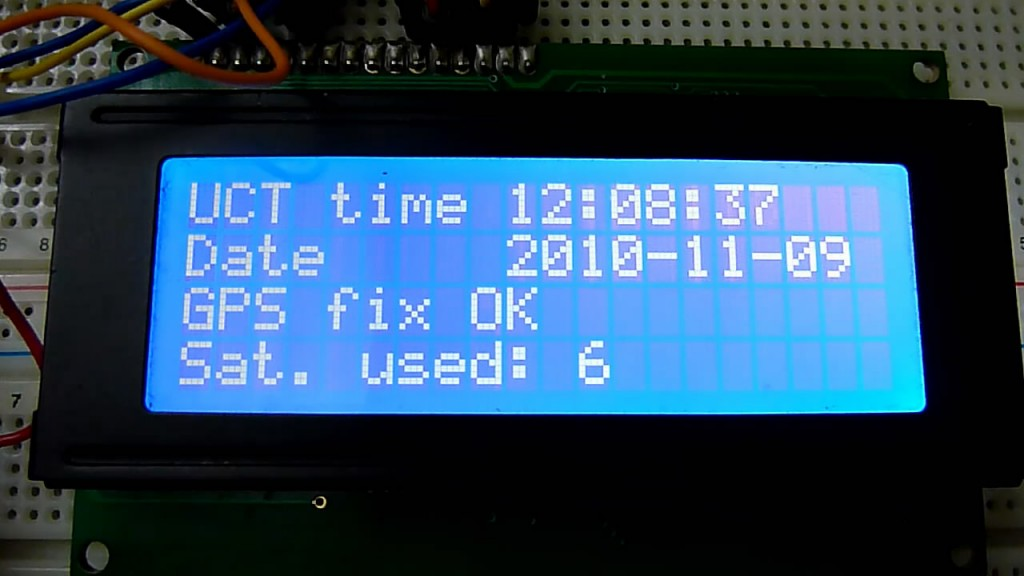GPS for accurate synchronization and  position measurement must use precise clock, so GPS satellites are equipped with atomic clocks. Clock accuracy is amazing ± 1 second in 1 million years. Using GPS module is available not only acquire position, speed, bet also time and date, so in this post I’ll explain how to do it.

GPS clock consist of old Sirf II GPS module, MAX 232, Arduino Mega and LCD display (Hitachi HD44780).

Sirf II module has RS-232 interface for communication and it can be  connected to PC Com port. Atmega in Arduino board has  UART interface. RS-232 basically is the same UART, only zeros and ones voltage levels are different. To match levels MAX232 driver is used. Today’s GPS modules have UART port, so there isn’t any need for MAX232.

Arduino in this project doesn’t have clock function it just pass time and date from GPS module to display. It works that way because GPS module has internal RTC(Real time clock) it’s not accurate, but it is synchronized to GPS system.

As you can see from video GPS module RTC is sychronised before GPS fix happens, but GPS fix is only one indicator that shows that clock is synchronised.

# Examples of using Arduino/Atmega 16 bit hardware timer for digital clock

Arduino Mega with Atmega 1280 has four 16 bit timers, that could be used for various purposes, like time/frequency measurement, control with precise timing, PWM generation. Today I hope to explain how to use timer for clocks, timers (countdown) and other things, where You need µCPU to perform some tasks after precise period of time. I’ll give You two examples:

• Pseudo 1 second timer
• Real 1 second timer

How counter works? It is simple independent  16 bit accumulator, which value increases by 1 at clock cycle. 16 bit means that maximum counter’s value is 65536. When this value is reached counter starts counting from 0 again and gives hardware interrupt. Counter value could be changed any time. This is normal counter mode, Atmega 1280 offers total 14 operating modes.

Pseudo 1 second timer (1.048576 s)

# 4 digits, 7 segments LED display multiplexing with Arduino

Last time I showed You how to control 1 digit 7 segment LED display with Arduino. This time it’s not 1, but 4 digits. To connect 1 digit to Arduino we had to use 8 ports, so to connect 4 digits we need to have 4×8=32? Not necessary. Where is a way to use much less ports, it’s called multiplexing. Using multiplexing at one time only one digit is active(e.g. for 2ms). All digits is turned on is serial, but because human’s eye is inert we have illusion, that all digits are lighting at same time.

As You can see form schematic bellow with multiplexing implemented we required only 4 additional ports compared to 1 digit circuit, total – 12.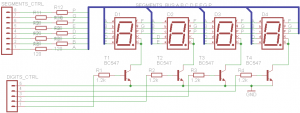Because at the same time only one digit will be on All 4 digits segments inputs are connected together. By connecting digits common cathodes to ground we are controlling which digit shall be turned on. Atmega 1280 µCPU port can drain(receive) maximum 40 mA current. If all one digits segments are on, we are having 20×8= 160 mA that is to much, so we can’t to connect common cathodes directly to Arduino ports.  Therefore I have used BC547 NPN transistors as switches. Transistor is opened, when positive voltage is applied at the base.

Let’s see the code.